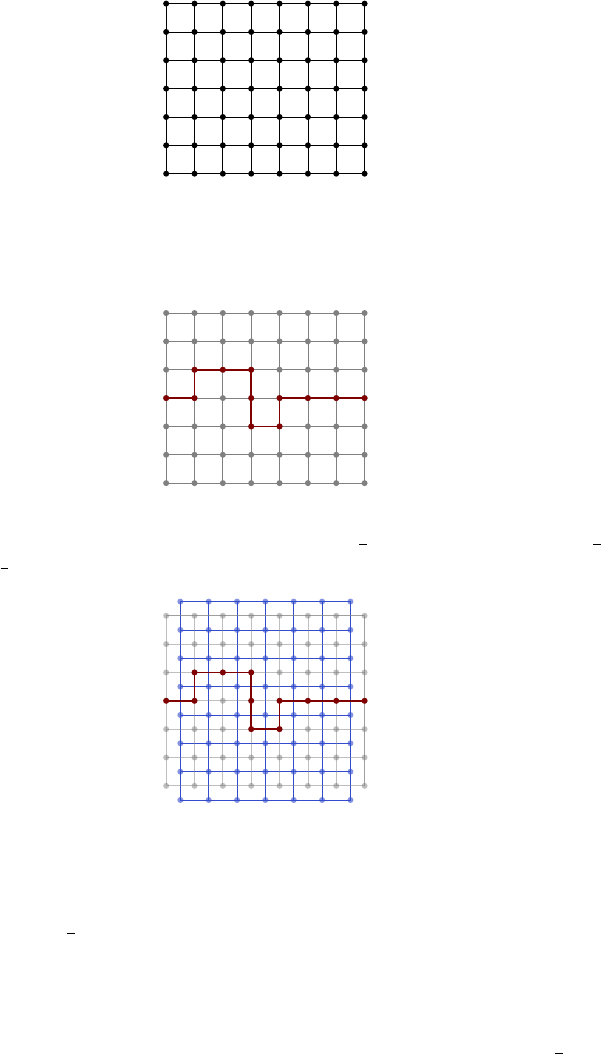0Introduction

III Percolation and Random Walks on Graphs0 Introduction
This course is naturally divided into two parts percolation, and random
walks on graphs. Percolation is one of the simplest models that experience phase
transition — an abrupt change in quantitative feature due to a continuous change
of a parameter. More sophisticated examples of percolation the boiling of water
and the loss of long-range correlation in magnets when temperature increases.
But we are not physicists. So let’s talk about percolation. For reasons that
become clear later, consider an n ×(n + 1) lattice connected by edges:
We now fix some
p
[0
,
1], and for each edge in the graph, we either keep it
or remove it with probability
p
. There are many questions we may ask. For
example, we may ask for the probability that there is a left-to-right crossing of
open edges.
For example, we have f
n
(0) = 0 and f
n
(1) = 1.
An interesting choice of
p
to consider is
p
=
1
2
. We can argue that
f
n
(
1
2
)
must be
1
2
, by symmetry. More precisely, consider the dual lattice:
Note that this lattice is isomorphic to the original lattice we were thinking about,
by applying a rotation. Now each edge in the dual lattice crosses exactly one edge
in the original lattice, and we can set the edge to be open iff the corresponding
edge in the original lattice is open. This gives rise to a percolation on the dual
lattice with p =
1
2
as well.
Now notice that that there is a left-right crossing of open edges in the original
lattice iff there is no top-bottom crossing in the dual graph. But since the dual
and original lattices are isomorphic, it follows that the probability that there is
a left-right crossing in the original lattice is equal to the probability that there
is no left-right crossing in the original lattice. So both of these must be
1
2
.
The ability to talk about the dual graph is a very important property that
is only true in 2 dimensions. In general, there are many things known for 2
dimensions via the dual, which do not generalize to higher dimensions.
The other topic we are going to discuss is random walks in graphs. In IB
Markov chains, and maybe IA Probability, we considered random walks on the
integer lattice
Z
d
. Here we shall consider random walks on any graph. We
shall mostly think about finite graphs, but we will also talk about how certain
results can be extended to infinite graphs. It turns out a rather useful way of